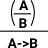# Maths - Exponent (Function) Types

A function type is sometimes called an exponent type. This is related to the way we usually use the term 'exponent' in algebra, for example in sets, the number of mappings from A to B is given by the number of elements in B to the power of the number of elements in A.

### Notation

 A->B or BA The function type is denoted A->B or BA. The second tents to be used for exponents in category theory. λ x . b(x) A function ( x -> b(x)) can be notated using lambda symbol: λ x . b(x) using '.' to seperate input parameter from output. M[x/N] Substitution The expression M in which every x is replaced by N

### Function Type

ref ncatlab

 In type theory a function type can be constructed from(deconstructed to) an expression.This requires the concept of substitution.

Usually defined as a 'negative type', that is we define it by how it is used (applying a function).

We can think of the type A->B as either,

• All the possible functions from type A to type B (a homset).
• Object of type B parameterised by an variable of type A.

I find it easier to understand the introduction and elimination rules in terms of the second interpretation.

 To introduce a term of type A->B we need a free variable in A and a term of type B containing x.### Rules

Types are defined by their formation, term introduction, term eliminator and computation rules:

Function Type

formation rule

Given any two types A and B we can form a function type A->BA:TypeB:TypeA->B : Type

term introduction rule

assume a implies b

(given a proof of A we get a proof of B)

lambda abstraction:

 Γ a:Ab:B ΓB:Type Γλa.b : A -> B

term eliminator

Modus Ponens

application:

 ΓA->B:Type Γa:A Γf(a) : B

computation rule

substitution - in this case of a for x.

( λ x . b(x)) (a) ->β b(a)

we reduce this by substitution.

b[a/x]

Local completeness rule

eta reduction rule

M:A->B ->η λ x. M x

So function terms are introduced using the lambda abstraction. This is a binder which captures a variable (see lambda calculus page).

We eliminate a function term by applying it. In logic this is known as 'modus ponens' that is: given A and a function from A to B we can imply B.

So the term introduction rule binds a variable and the term eliminator removes that variable.

### β-reduction and η-reduction

 An element of A->B can be represented as λ a.Φ `λ a.Φ : A->B` β-reduction The second form allows us to change the name of the variable by substitution. Replaces a bound variable in a function body with a function argument - inlining. ```(λ a.Φ) a = Φ (λ a.Φ) x = [a/x]Φ``` η-reduction `f = (λ x . f(x))`

#### β Reduction

 (λx.M) N M[N/x]
Function evaluation is called β-reduction. This is done by substitution.

#### β Equality

Two terms are β-equal is one β-reduces to the other one.

M =βN

if there are terms M0 to Mn such that M0M, MnN and for all i such that 0 ≤i<n

either: Mi =βMi+1 or Mi+1 =βMi

#### β-Normal Form

• M is in β-normal form if M does not contain any redux
• M is weakly normalising if there is an N in β-normal form such that M -> βN
• M is strongly normalising if there are no infinite reduction paths starting from M

## Idris Examples

 Constructor ```constructExp : Int -> String constructExp = \ x => prim__toStrInt x``` ```*typeTheory> constructExp \x => prim__toStrInt x : Int -> String```

 Deconstructor ```applyFn : (t1 -> t2) -> t1 -> t2 applyFn f v = f v``` ```*typeTheory> applyFn prim__toStrInt 3 "3" : String ```

#### Idris

from : Idris-dev/libs/prelude/Prelude/Basics.idr

```||| Function application.
apply : (a -> b) -> a -> b
apply f a = f a
```

We can implement in Idris like this:

 We create anominous lambda like this and apply it like this. ```Idris> \x => prim__toStrInt x \x => prim__toStrInt x : Int -> String Idris> (\x => prim__toStrInt x) 3 "3" : String```

## Logic

In logic this structure corresponds to 'implication'.

There is an interpretaion of type theory (known as Curry-Howard) that has the same structure as intuitional logic. In this interpretation an element (term) of a type is a proof of that type.

 Implication is a bit like taking a whole rule, putting brackets round it and using it as a premise for another rule.### Implication and Modus Ponens

This is discussed further on the propositional logic page.

Introduction Elimination
(modus ponens)
 [A] ... B A->B
(=>I)
 A A->B B
(=>E)

Alternatively we can represent modus ponens using context () as follows:

 C(B => D) C /\ BD

### λ-calculus

(see page here)

Here is a reminder of the notation:

 lambda variable dot (separator) expression λ x . 2*x+1

Note: although I have used an arithmetic expression above, λ-terms are usually defined in a more abstract way, in the extreme case every term is a function.

T ::= V | (T T) | (λV.T)

where

• V is a variable (a symbol taken from an infinite set of symbols).
• (T T) juxtaposition is function application, different from T(T) notation.

### Next Steps

Other types are discussed on the following pages:

Where I can, I have put links to Amazon for books that are relevant to the subject, click on the appropriate country flag to get more details of the book or to buy it from them.Computation and Reasoning - This book is about type theory. Although it is very technical it is aimed at computer scientists, so it has more discussion than a book aimed at pure mathematicians. It is especially useful for the coverage of dependant types.